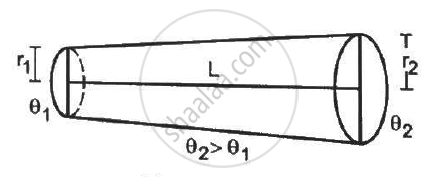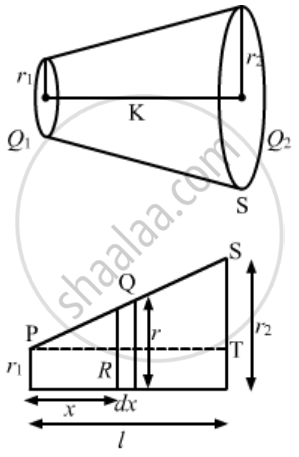Share

# Find the Rate of Heat Flow Through a Cross Section of the Rod Shown in Figure (28-e10) (θ2 > θ1). Thermal Conductivity of the Material of the Rod is K. - Physics

ConceptThermal Expansion of Solids

#### Question

Find the rate of heat flow through a cross section of the rod shown in figure (28-E10) (θ2 > θ1). Thermal conductivity of the material of the rod is K.#### SolutionWe can infer from the diagram that ΔPQR is similar to ΔPST.
So, by the property of similar triangles,
x/l = (r - r_1)/(r_2 - r_1)
⇒ (r_2 - r_1) x/l = r - r_1
⇒ r = r_1 + ( r_2 - r_1 )  x/l
Let ;
(r_2 - r_1)/l = a
⇒ r = ax + r_1................(i)

Thermal resistance is given by

dR = dx/(K.A)

⇒ dR = (dx)/(K.pir^2]

dR = (dx)/(K.pi (ax + r_1)^2

$\int\limits_0^R$ dR = 1/(kpi)  $\int\limits_0^l$ dx/(ax + r_1)

R  = -(1)/(Kpi) [(1)/(ax + r_1)]_0^6

R =  -(1)/(aKpi)[(1)/(al + r_1) -1/(r1)]

R = -(1)/(aKpi) [ 1/(((r_2 -r_1)/l) l + r_1) - 1/r_1]

R = - 1/ (aKpi)[(1)/(r_2)-(1)/(r_1)]

R = -(1)/(Kpir_1r_2)

Rate of flow of heat = (DeltaQ)/R

⇒ q = ( Q_2 - Q_1)/l Kpir_1r_2`

Is there an error in this question or solution?

#### APPEARS IN

Solution Find the Rate of Heat Flow Through a Cross Section of the Rod Shown in Figure (28-e10) (θ2 > θ1). Thermal Conductivity of the Material of the Rod is K. Concept: Thermal Expansion of Solids.
S